Examples

Practical Geometry
Serial order wise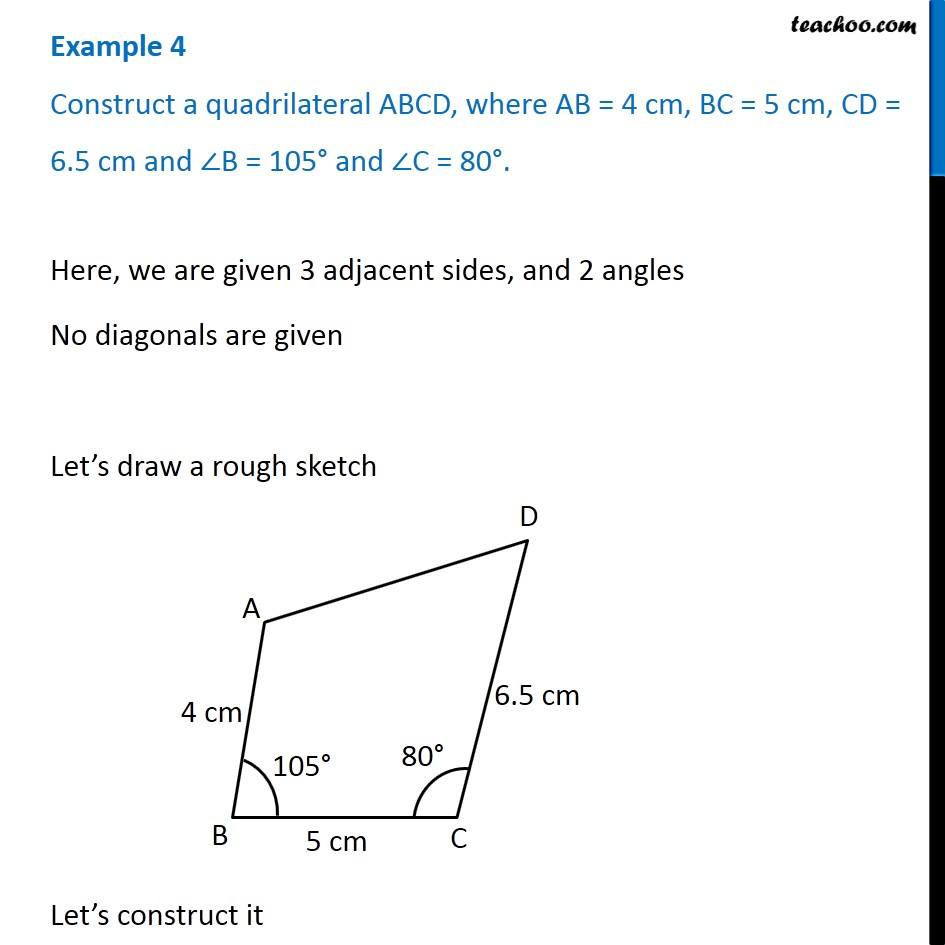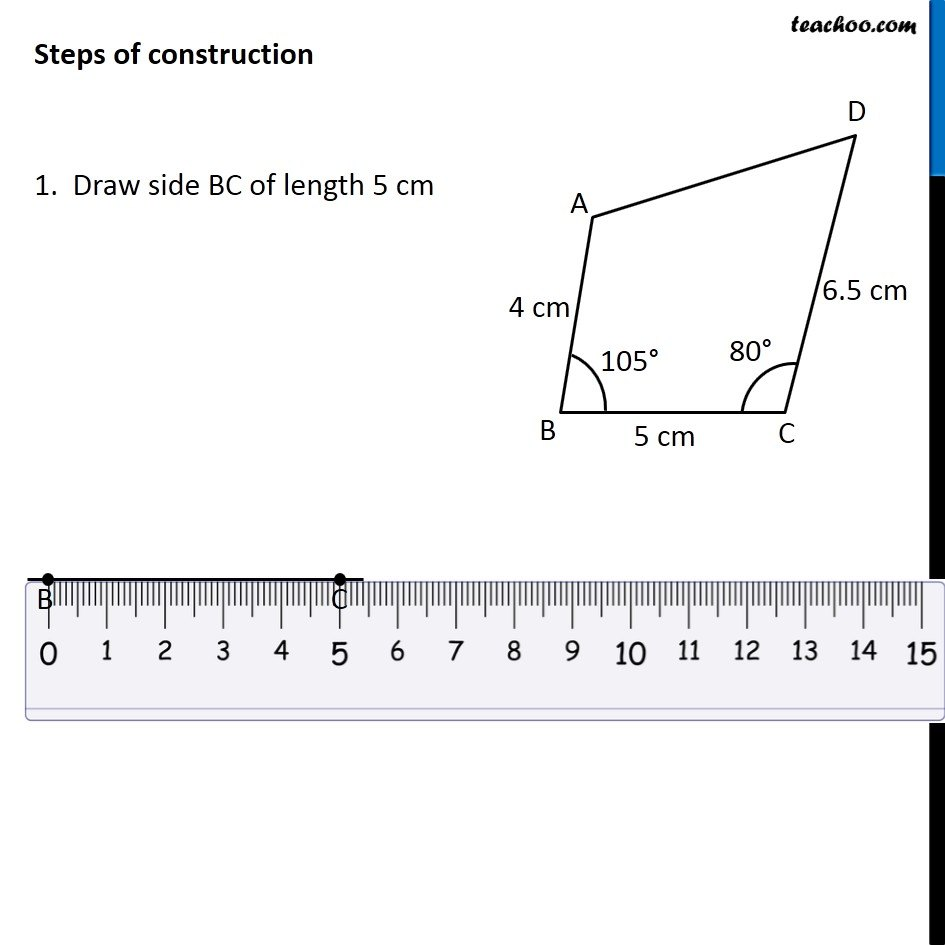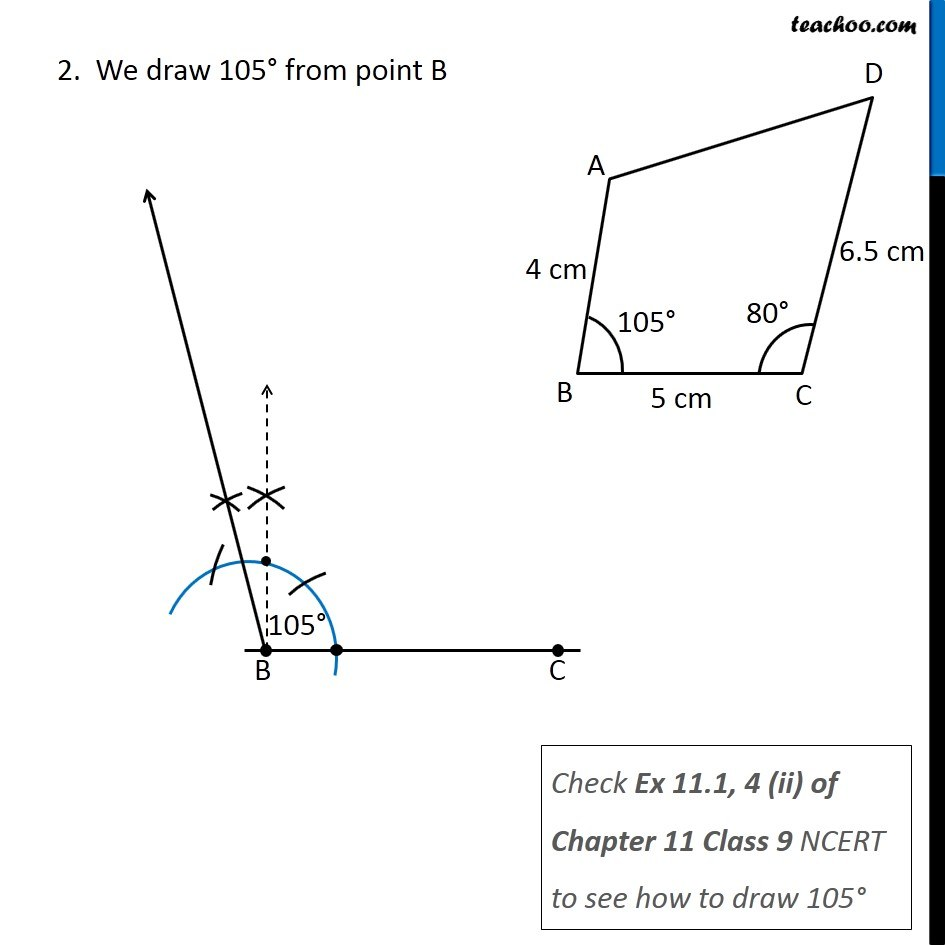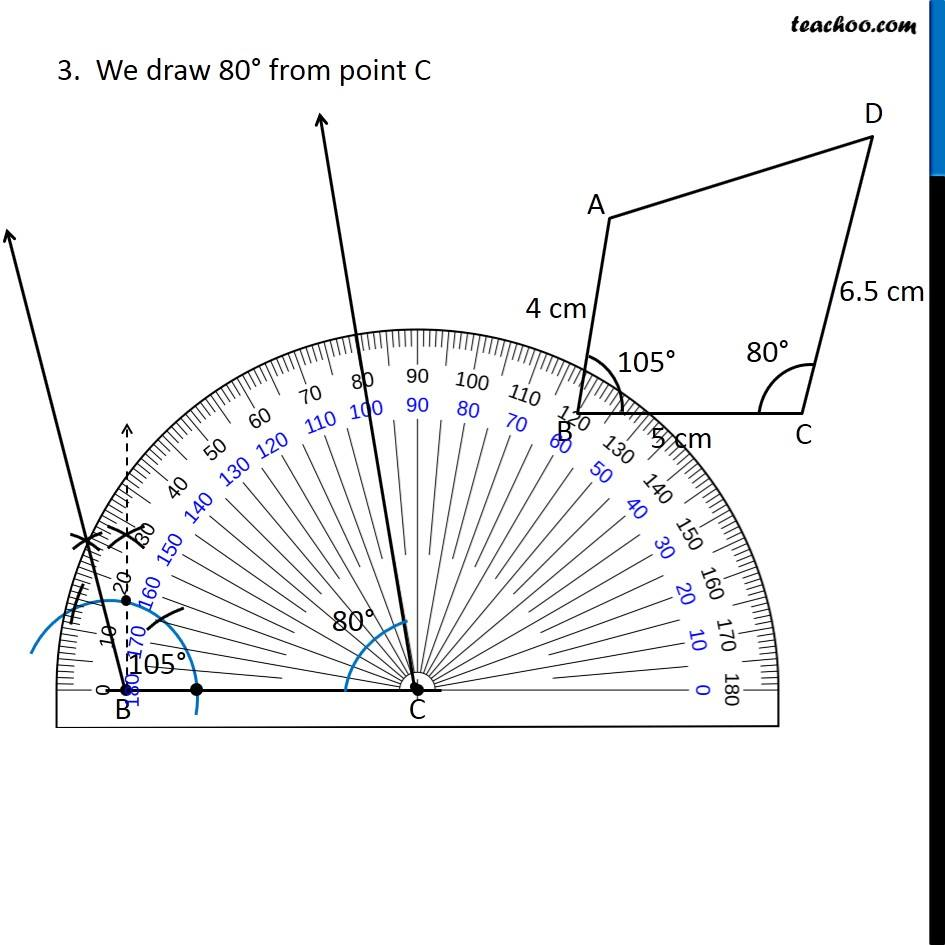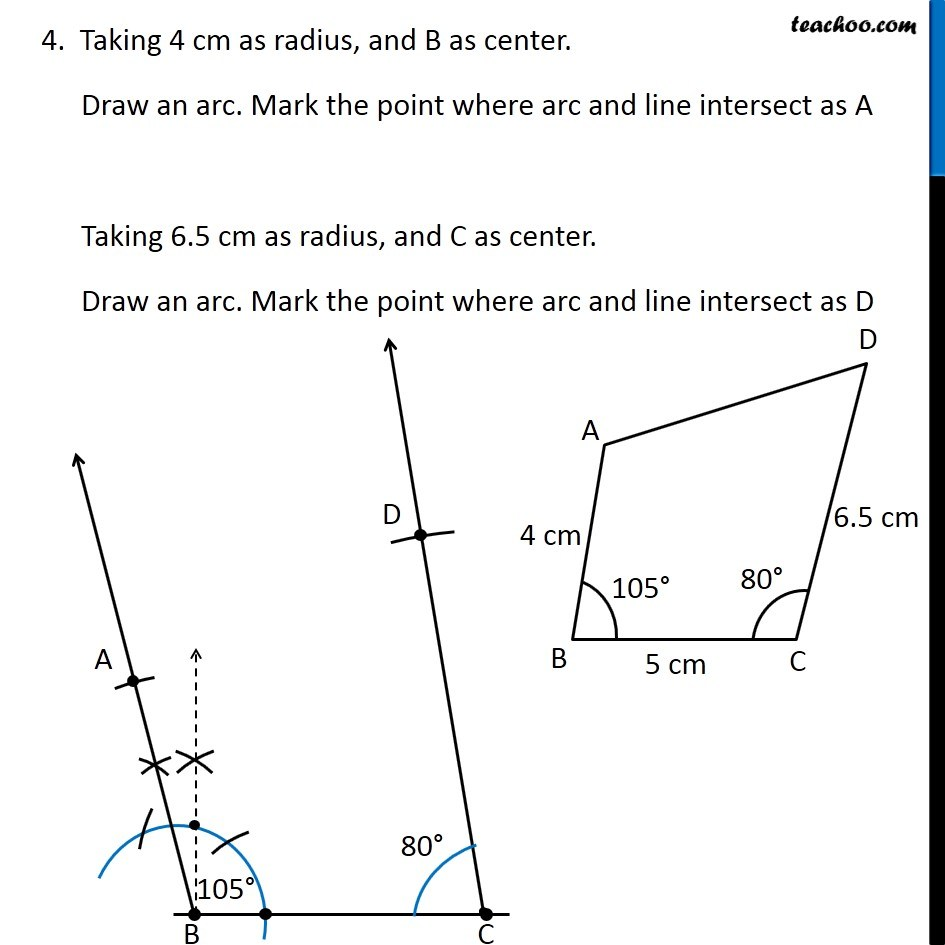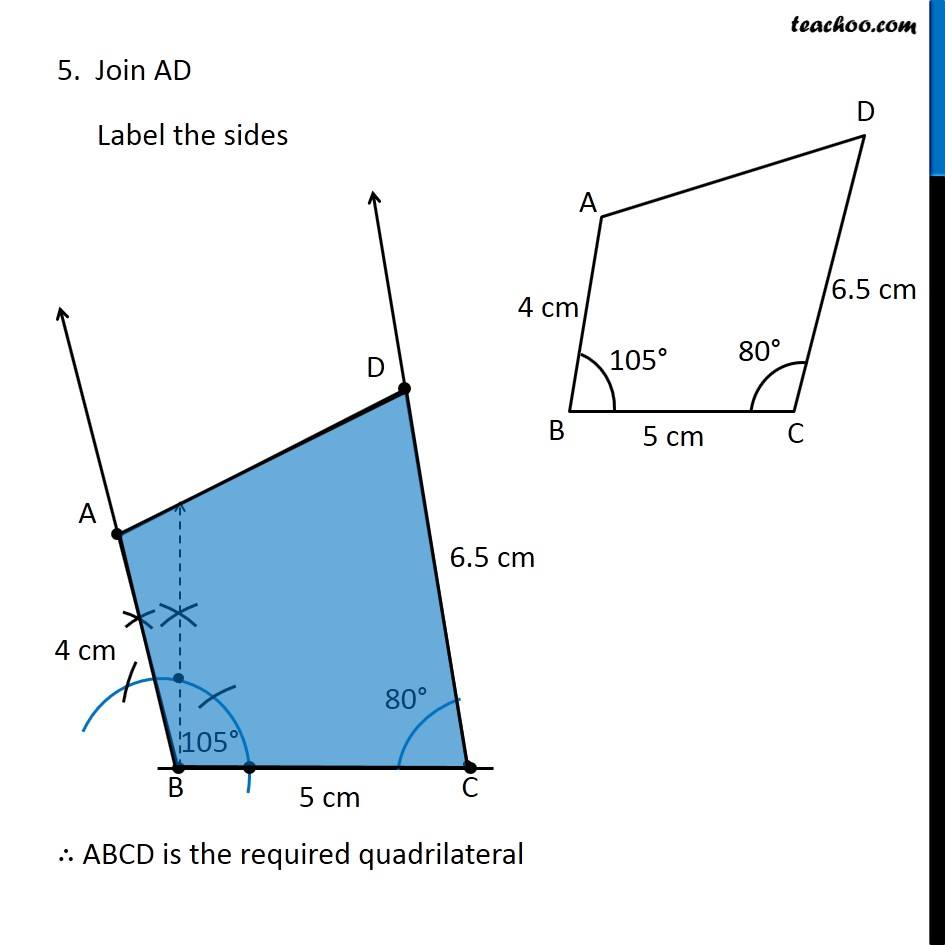Learn in your speed, with individual attention - Teachoo Maths 1-on-1 Class

### Transcript

Example 4 Construct a quadrilateral ABCD, where AB = 4 cm, BC = 5 cm, CD = 6.5 cm and ∠B = 105° and ∠C = 80°. Here, we are given 3 adjacent sides, and 2 angles No diagonals are given Let’s draw a rough sketch Let’s construct it Steps of construction 1. Draw side BC of length 5 cm 2. We draw 105° from point B Check Ex 11.1, 4 (ii) of Chapter 11 Class 9 NCERT to see how to draw 105° 3. We draw 80° from point C 4. Taking 4 cm as radius, and B as center. Draw an arc. Mark the point where arc and line intersect as A Taking 6.5 cm as radius, and C as center. Draw an arc. Mark the point where arc and line intersect as D 5. Join AD Label the sides